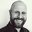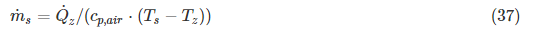Question-and-Answer Resource for the Building Energy Modeling Community
Get started with the Help page

# Ideal heating: Calculation thermal energy

I implemented a simple room model in matlab (incl. weather data) - output is the roomTemperature. Now i want to calculate the ideal thermal energy which is neede for a constant room temperature. Is it common to calculate it with constant mass flow or constant supply-temperature?

Given: The room-volume, the room temperature

I want to calculate: The power of an idealHeater (each timestep)

$Q = c \times m \times \Delta T$ --> ??

edit retag close merge delete

Sort by » oldest newest most votedCalculation 37 in the ideal air loads engineering reference suggests that at least in EnergyPlus it's a fixed supply temperature, and the mass flow is the calculated value.

Calculate supply air mass flow rate required to meet zone sensible load at the applicable (heating or cooling) supply temperature limit (Tmax,heating or Tmin,cooling)more

Thank you very much: If I would just want to know, how much energy I need for heating from the actual room-temperature to a given set point temperature, would it be possible to calculate it that way: Q = VolumeRoom * density * cp * (Tset – Troom). Then calculate the thermal power for a given internal gain?